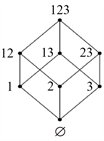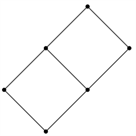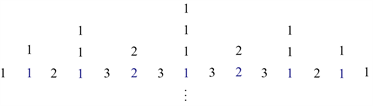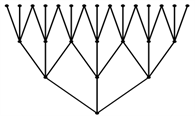#### 期刊菜单

Stern偏序集的flag f和flag h向量的性质研究
The flag f and flag h Vectors of the Stern’s Poset
DOI: 10.12677/AAM.2021.108273, PDF , HTML, XML, 下载: 320  浏览: 460

Abstract: The Stern’s poset is one kind of partially ordered set with great importance and interest in Combinatorics, which plays a unifying role in combinatorial counting. In this paper, we study the flag f and flag h vectors of the Stern’s poset from the viewpoint of counting order ideal, and then give the specific expression formulas.

1. 引言

1) 自反性：对所有P中元素x都有 $x\le x$

2) 反对称性：如果 $x\le y$$y\le x$ 则有 $x=y$

3) 传递性：如果 $x\le y$$y\le z$ 则有 $x\le z$Figure 1. ${\mathcal{B}}_{3}$Figure 2. ${D}_{12}$

Pascal三角是数学中非常著名的三角，它的前几项如图3所示。Figure 3. Pascal triangleFigure 4. Stern’s triangleFigure 5. Stern’s poest

R. Stanley定义了Stern偏序集并证明了对一个固定整数 $r\ge 1$，第n行元素的第n次幂和 ${u}_{r}\left(n\right)$ 满足常系数线性递归 。通过对Stern偏序集主理想的计数可以得到许多组合序列。

2. Stern偏序集的flag f和flag h向量

$\stackrel{˜}{f}\left({i}_{j}\right)={2}^{{i}_{j}+1}-1,\text{\hspace{0.17em}}\stackrel{˜}{f}\left(S\right)=\stackrel{˜}{f}\left({i}_{1}\right)\stackrel{˜}{f}\left({i}_{2}-{i}_{1}\right)\stackrel{˜}{f}\left({i}_{3}-{i}_{2}\right)\cdots \stackrel{˜}{f}\left({i}_{t}-{i}_{t-1}\right)$

$\stackrel{˜}{f}\left({i}_{j},{i}_{k}\right)$ 是秩为 ${i}_{j}$ 层和秩为 ${i}_{k}$ 层之间的最大链个数，而秩为 ${i}_{j}$ 层每个元素到秩为 ${i}_{k}$ 层均有 $\left({2}^{{i}_{k}-{i}_{j}+1}-1\right)$ 条最大链，即 $\stackrel{˜}{f}\left({i}_{k}-{i}_{j}\right)$ 条最大链，而秩为 ${i}_{j}$ 层共有 $\stackrel{˜}{f}\left({i}_{j}\right)$ 个元素，故 $\stackrel{˜}{f}\left({i}_{j},{i}_{k}\right)=\stackrel{˜}{f}\left({i}_{j}\right)\stackrel{˜}{f}\left({i}_{k}-{i}_{j}\right)$

$\stackrel{˜}{h}\left({i}_{j}\right)={2}^{{i}_{j}+1}-2,\stackrel{˜}{h}\left(S\right)=\stackrel{˜}{h}\left({i}_{1}\right)\stackrel{˜}{h}\left({i}_{2}-{i}_{1}-1\right)\stackrel{˜}{h}\left({i}_{3}-{i}_{2}-1\right)\cdots \stackrel{˜}{h}\left({i}_{t}-{i}_{t-1}-1\right)$.

$\stackrel{˜}{h}\left({i}_{j}\right)=-1+\stackrel{˜}{f}\left({i}_{j}\right)=-1+{2}^{{i}_{j}+1}-1={2}^{{i}_{j}+1}-2$ (2.1)

$\stackrel{˜}{h}\left({i}_{j},{i}_{k}\right)=1-\stackrel{˜}{f}\left({i}_{j}\right)-\stackrel{˜}{f}\left({i}_{k}\right)+\stackrel{˜}{f}\left({i}_{j},{i}_{k}\right)=\stackrel{˜}{h}\left({i}_{j}\right)\stackrel{˜}{h}\left({i}_{k}-{i}_{j}-1\right)$

$\stackrel{˜}{h}\left({S}_{1}\right)=\stackrel{˜}{h}\left({i}_{1}\right)\stackrel{˜}{h}\left({i}_{2}-{i}_{1}-1\right)\stackrel{˜}{h}\left({i}_{3}-{i}_{2}-1\right)\cdots \stackrel{˜}{h}\left({i}_{t-1}-{i}_{t-2}-1\right)$ (2.2)

$\begin{array}{c}\stackrel{˜}{h}\left(S\right)={\left(-1\right)}^{t}+{\left(-1\right)}^{t-1}\underset{1\le j\le t}{\sum }\stackrel{˜}{f}\left({i}_{j}\right)+{\left(-1\right)}^{t-2}\underset{j

$\begin{array}{l}{\left(-1\right)}^{t-1}\stackrel{˜}{f}\left({i}_{t}\right)+{\left(-1\right)}^{t-2}\underset{j=1}{\overset{t-1}{\sum }}\stackrel{˜}{f}\left({i}_{j},{i}_{t}\right)+\cdots +\stackrel{˜}{f}\left(S\right)\\ =\stackrel{˜}{h}\left({S}_{1}\right)\left(1+\stackrel{˜}{h}\left({i}_{t}-{i}_{t-1}-1\right)\right)=\stackrel{˜}{h}\left({S}_{1}\right)\stackrel{˜}{f}\left({i}_{t}-{i}_{t-1}-1\right)\end{array}$ (2.3)

$\stackrel{˜}{h}\left(S\right)=-\stackrel{˜}{h}\left({S}_{1}\right)+\stackrel{˜}{h}\left({S}_{1}\right)\left(1+\stackrel{˜}{h}\left({i}_{t}-{i}_{t-1}-1\right)\right)=\stackrel{˜}{h}\left({S}_{1}\right)\stackrel{˜}{h}\left({i}_{t}-{i}_{t-1}-1\right)$

$\stackrel{˜}{f}\left({i}_{t}\right)-\stackrel{˜}{f}\left({i}_{j},{i}_{t}\right)=-\stackrel{˜}{h}\left({i}_{j}\right)\stackrel{˜}{f}\left({i}_{t}-{i}_{j}-1\right)=-2\stackrel{˜}{f}\left({i}_{j}-1\right)\stackrel{˜}{f}\left({i}_{t}-{i}_{j}-1\right)$ (2.4)

$\stackrel{˜}{f}\left({i}_{1},\cdots ,{i}_{r},{i}_{t}\right)-\stackrel{˜}{f}\left({i}_{1},\cdots ,{i}_{r},{i}_{t-1},{i}_{t}\right)=-2\stackrel{˜}{f}\left({S}_{t-r}\right)\stackrel{˜}{f}\left({i}_{t}-{i}_{r}-1\right)\stackrel{˜}{f}\left({i}_{t}-{i}_{t-1}-1\right)$ (2.5)

$\begin{array}{c}\stackrel{˜}{f}\left({i}_{t}\right)-\stackrel{˜}{f}\left({i}_{j},{i}_{t}\right)={2}^{{i}_{t}+1}-1-\left({2}^{{i}_{j}+1}-1\right)\left({2}^{{i}_{t}-{i}_{j}+1}-1\right)\\ =-\left({2}^{{i}_{j}+1}-2\right)\left({2}^{{i}_{t}-{i}_{j}}-1\right)\\ =-\stackrel{˜}{h}\left({i}_{j}\right)\stackrel{˜}{f}\left({i}_{t}-{i}_{j}-1\right)\\ =-2\stackrel{˜}{f}\left({i}_{j}-1\right)\stackrel{˜}{f}\left({i}_{t}-{i}_{j}-1\right)\end{array}$

$\begin{array}{l}\stackrel{˜}{f}\left({i}_{1},\cdots ,{i}_{r},{i}_{t}\right)-\stackrel{˜}{f}\left({i}_{1},\cdots ,{i}_{r},{i}_{t-1},{i}_{t}\right)\\ =\stackrel{˜}{f}\left({S}_{t-r}\right)\stackrel{˜}{f}\left({i}_{t}-{i}_{r}\right)-\stackrel{˜}{f}\left({S}_{t-r}\right)\stackrel{˜}{f}\left({i}_{t-1}-{i}_{r}\right)\stackrel{˜}{f}\left({i}_{t}-{i}_{t-1}\right)\\ =\stackrel{˜}{f}\left({S}_{t-r}\right)\left[{2}^{{i}_{t}-{i}_{r}+1}-1-\left({2}^{{i}_{t-1}-{i}_{r}+1}-1\right)\left({2}^{{i}_{t}-{i}_{t-1}+1}-1\right)\right]\\ =-\stackrel{˜}{f}\left({S}_{t-r}\right)\left({2}^{{i}_{t-1}-{i}_{r}}-2\right)\left({2}^{{i}_{t}-{i}_{t-1}+1}-2\right)\\ =-2\stackrel{˜}{f}\left({S}_{t-r}\right)\left({2}^{{i}_{t-1}-{i}_{r}}-1\right)\left({2}^{{i}_{t}-{i}_{t-1}}-1\right)\\ =-2\stackrel{˜}{f}\left({S}_{t-r}\right)\stackrel{˜}{f}\left({i}_{t}-{i}_{r}-1\right)\stackrel{˜}{f}\left({i}_{t}-{i}_{t-1}-1\right)\end{array}$ $\square$

$\begin{array}{l}{\left(-1\right)}^{t-1}\left[\stackrel{˜}{f}\left({i}_{t}\right)-\stackrel{˜}{f}\left({i}_{t-1},{i}_{t}\right)\right]+{\left(-1\right)}^{t-2}\underset{j

${\left(-1\right)}^{t}2\stackrel{˜}{f}\left({i}_{t-1}-1\right)+{\left(-1\right)}^{t-1}2\underset{j (2.6)

$\left(2.6\right)=\stackrel{˜}{h}\left( S 1 \right)$

$\begin{array}{c}\left(2.6\right)=2\stackrel{˜}{f}\left({i}_{t-1}-1\right)\stackrel{˜}{h}\left({S}_{2}\right)+{\left(-1\right)}^{t}\underset{j

$\begin{array}{l}{\left(-1\right)}^{t}\underset{j (2.7)

$\begin{array}{l}{\left(-1\right)}^{t-1}\underset{j

$\begin{array}{c}\left(2.7\right)={2}^{{i}_{t-1}-{i}_{t-3}}\stackrel{˜}{f}\left({i}_{t-3}\right)\stackrel{˜}{h}\left({S}_{3}\right)+{2}^{{i}_{t-1}-{i}_{t-2}}\stackrel{˜}{h}\left({i}_{t-2}\right)\left[{\left(-1\right)}^{t}\stackrel{˜}{f}\left({i}_{t-2}\right)+{\left(-1\right)}^{t-1}\underset{j $\square$

$\begin{array}{c}\left(2.6\right)=2\stackrel{˜}{f}\left({i}_{t-1}-1\right)\stackrel{˜}{h}\left({S}_{2}\right)-{2}^{{i}_{t-1}-{i}_{t-2}}\stackrel{˜}{f}\left({i}_{t-2}\right)\stackrel{˜}{h}\left({S}_{2}\right)\\ =\left[2\stackrel{˜}{f}\left({i}_{t-1}-1\right)-{2}^{{i}_{t-1}-{i}_{t-2}}\stackrel{˜}{f}\left({i}_{t-2}\right)\right]\stackrel{˜}{h}\left({S}_{2}\right)\\ =\left({2}^{{i}_{t-1}-{i}_{t-2}}-2\right)\stackrel{˜}{h}\left({S}_{2}\right)\\ =\stackrel{˜}{h}\left({i}_{t-1}-{i}_{t-2}-1\right)\stackrel{˜}{h}\left({S}_{2}\right)\\ =\stackrel{˜}{h}\left({S}_{1}\right)\end{array}$ $\square$

3. 结论

Stern偏序集的定义由R. Stanley给出，我们根据Stern偏序集的性质和结构特点对其flag f和flag h向量的公式进行归纳。并应用牟丽丽给出的Hexagonal偏序集的flag f和flag h向量公式的方法，给出了Stern偏序集的flag f和flag h向量的具体表达式。

  Rotr, C. (1964) On the Foundations of Combinatorial Theory I, Theory of Mobiousfunctions. Zeitschrift für Wahrscheinlichkeitstheorie und verwandte Gebiete, 2, 340-368. https://doi.org/10.1007/BF00531932  Stanley, R. (1997) Enumerative Combinatorics Vol. 1. Cambridge University Press, Cambridge. https://doi.org/10.1017/CBO9780511805967  Stanley, R. (2020) Some Linear Recurrences Motivated by Stern’s Diatomic Array. The American Mathematical Monthly, 127, 99-111. https://doi.org/10.1080/00029890.2020.1677104  Stanley, R. (2012) Enumerative Combinatorics. 2nd Edition, Vol. 1. Cambridge University Press, Cambridge.  Nyman, K. and Swartz, E. (2004) Inequalities for the h-Vectors and Flag h-Vectors of Geometric Lattices. Discrete & Computational Geometry, 32, 533-548. https://doi.org/10.1007/s00454-004-1137-z  Schweig, J. (2010) Convex-Ear Decompositions and the Flag H-Vector. Electronic Journal of Combinatorics, 18, 4. https://doi.org/10.37236/491  Mu, L. (2020) Some Combinatorial Properties of Hexagonal Poset. Contributions to Discrete Mathematics, 15, 175-182.